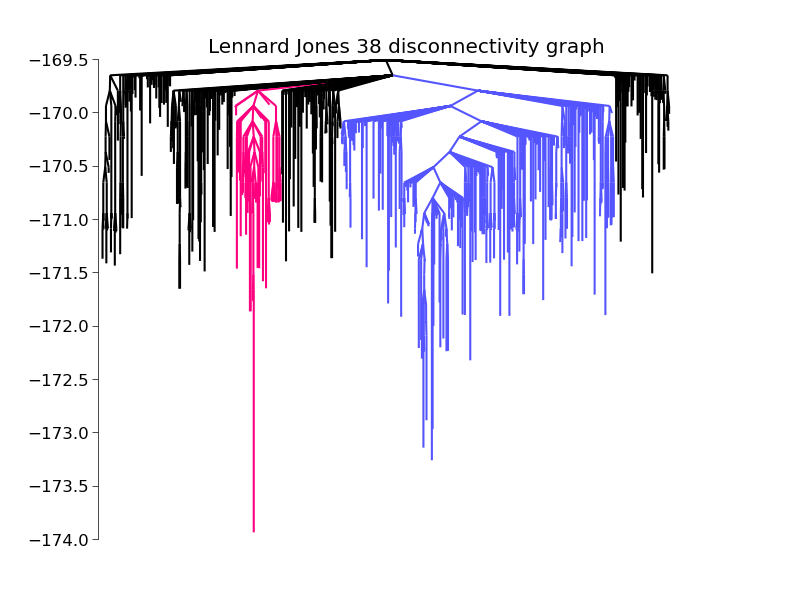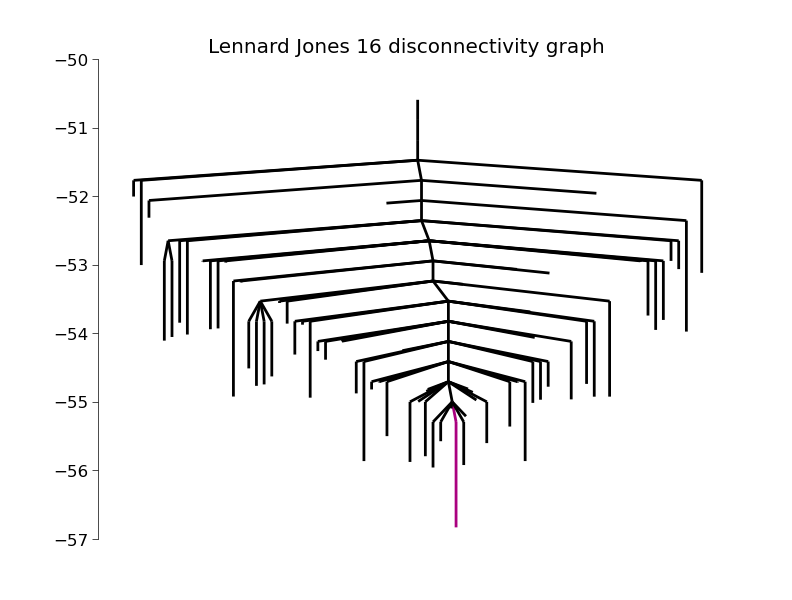# Disconnectivity Graphs¶

Disconnectivity graphs are an important way of visualizing the connectivity of an energy landscape. In a disconnectivity graph the graph is broken up at several levels of energy into groups of minima (basins) that are connected by transition states of energy less than that level.DisconnectivityGraph(graph[, minima, ...]) make a disconnectivity graph

In order to create a disconnectivity graph, you must have a database (storage), or a graph, of minima and transition states. These can be generated by using BasinHopping to find the minima and DoubleEndedConnect to find the transition states connecting them. Assuming you already have a database, here is how you turn it into a disconnectivity graph.

First you must convert the database to a networkx graph. This can be done with the function database2graph()

```from pele.landscape import database2graph
graph = database2graph(database)
```

Now we pass the graph to the class DisconnectivityGraph

```from pele.utils.disconnectivity_graph import DisconnectivityGraph
dg = DisconnectivityGraph(graph)
dg.calculate()
```

Finally, we plot it using matplotlib

```import matplotlib.pyplot as plt
dg.plot()
plt.show() # or simply dg.show()
```

See the matplotlib package for further options. I’ll just mention one here, saving the figure to a file.

```plt.savefig("tree.pdf")
```

## disconnectivity graph script¶

There is a handy script in pele which lets you create a disconnectivity graph from a database file directly from the command line. see the file

pele/scripts/make_disconnectivity_graph.py